Ex 10.4

Chapter 10 Class 11 Conic Sections
Serial order wise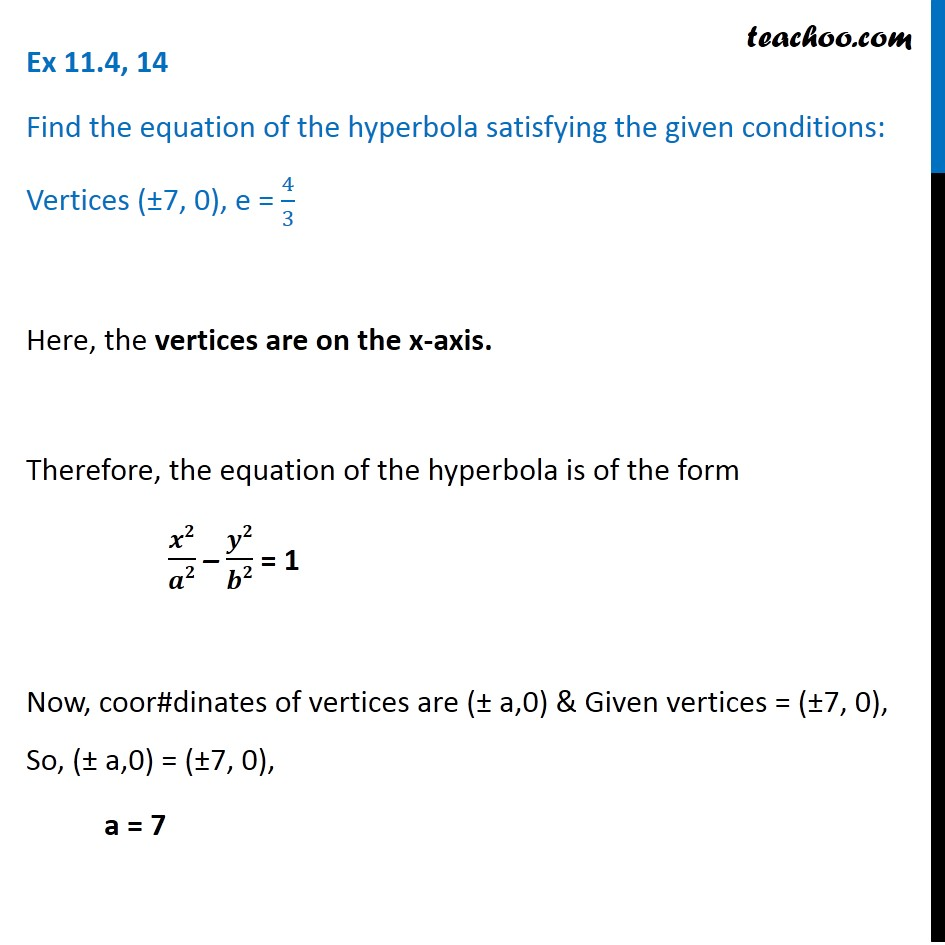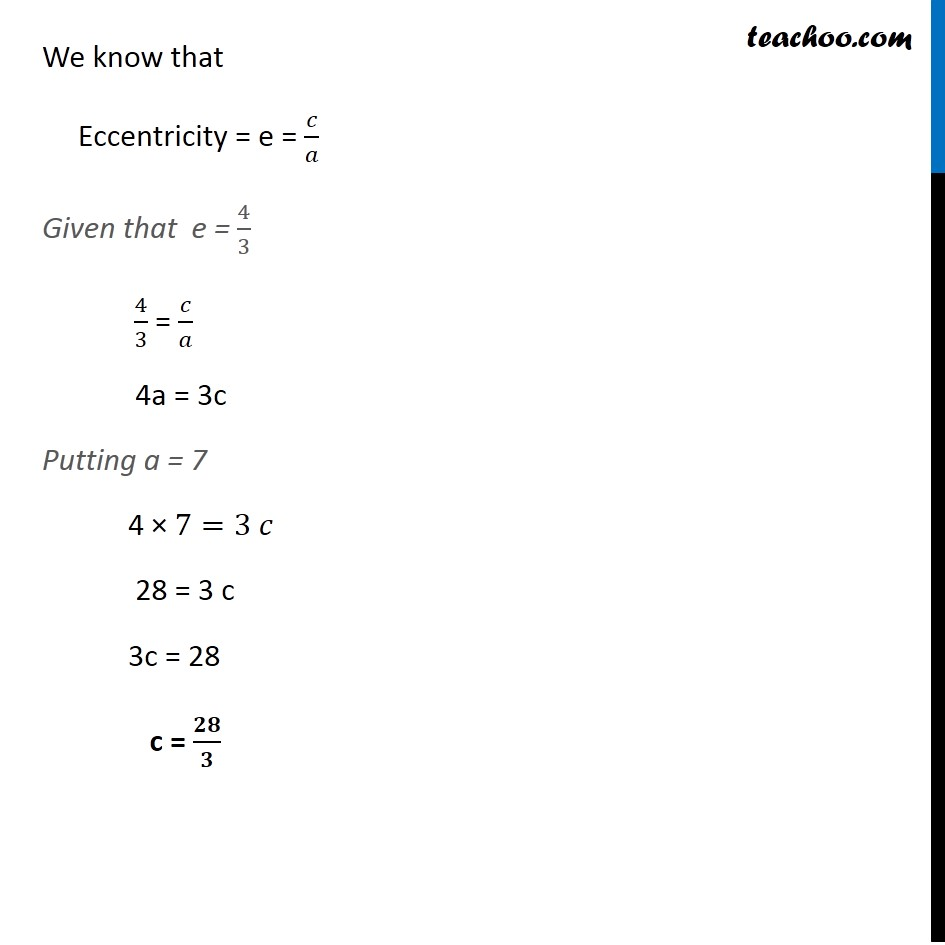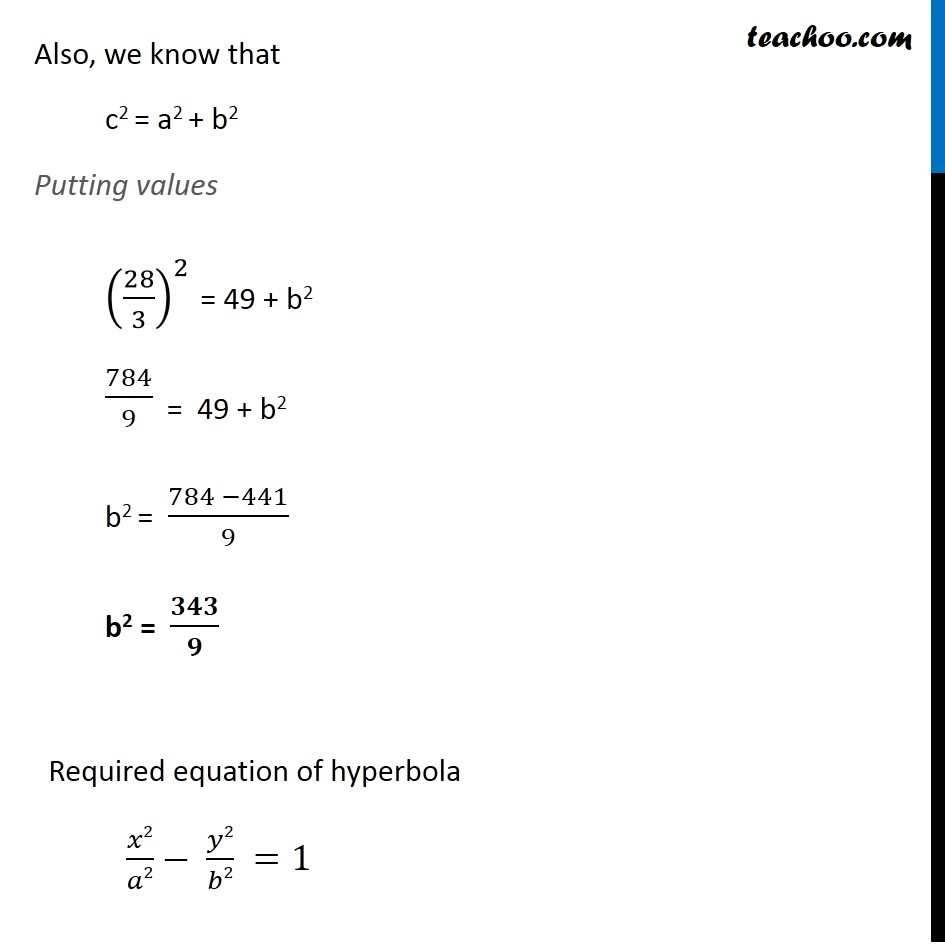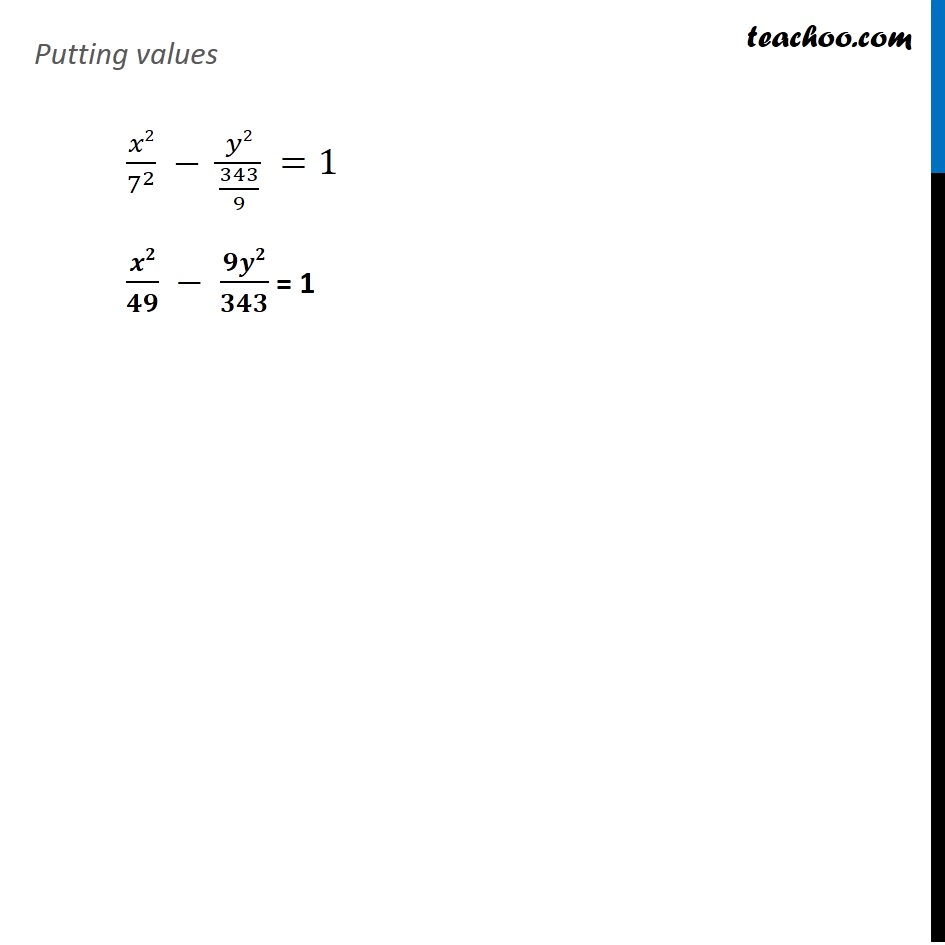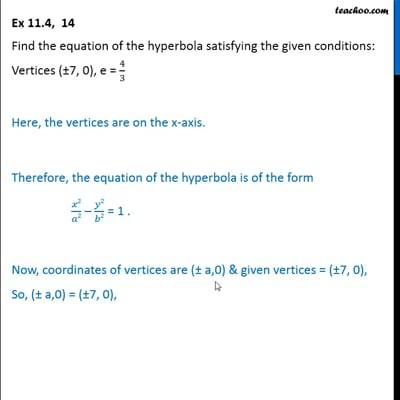This video is only available for Teachoo black users

Learn in your speed, with individual attention - Teachoo Maths 1-on-1 Class

### Transcript

Ex 10.4, 14 Find the equation of the hyperbola satisfying the given conditions: Vertices (±7, 0), e = 4/3 Here, the vertices are on the x-axis. Therefore, the equation of the hyperbola is of the form 𝒙𝟐/𝒂𝟐 – 𝒚𝟐/𝒃𝟐 = 1 Now, coor#dinates of vertices are (± a,0) & Given vertices = (±7, 0), So, (± a,0) = (±7, 0), a = 7 We know that Eccentricity = e = 𝑐/𝑎 Given that e = 4/3 4/3 = 𝑐/𝑎 4a = 3c Putting a = 7 4 × 7=3 𝑐 28 = 3 c 3c = 28 c = 𝟐𝟖/𝟑 Also, we know that c2 = a2 + b2 Putting values (28/3)^2 = 49 + b2 784/9 = 49 + b2 b2 = (784 −441)/9 b2 = 𝟑𝟒𝟑/𝟗 Required equation of hyperbola 𝑥2/𝑎2− 𝑦2/𝑏2 =1 Putting values 𝑥2/7^2 − 𝑦2/(343/9) =1 𝒙𝟐/𝟒𝟗 − 𝟗𝒚𝟐/𝟑𝟒𝟑 = 1